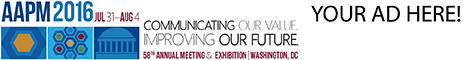# Program Information

## Tumor Control Probability Applied to Lung Cancer: A Statistical Test of the Independence of Voxel ResponseA Fontanella

## Presentations

#### TH-AB-304-3 (Thursday, July 16, 2015) 7:30 AM - 9:30 AM Room: 304

Purpose: Conventional radiotherapy planning uses simplified DVH-derived metrics (e.g. D99, D95) as surrogates for tumor control probability when evaluating plan quality. While this has the advantage of providing an easily derived predictor variable, such metrics fail to consider critical aspects of the underlying radiobiology. Counter to observed radiobiological effects, there is a prevailing assumption of tumor subvolume independence. We tested this by introducing a parameter to capture departures from independence using a modified metric, termed the “generalized tumor dose” (gTD).

Methods: For given dose distributions, cell survival was derived from a comprehensive biological model (Jeong, 2013 Phys. Med. Biol.). The gTD metric applied a generalized mean term, q, to subvolume survival values, with this term optimized according to its predictive power over the NSCLS cohort (N=316). Patients were treated 1.8-2.2 Gy/Fx in 28-39 Fxs (n=50), or 2.75 Gy/Fx in 24 fractions (n=266). Results were compared to other metrics according binary outcome and actuarial survival prediction.

Results: Fitting model parameters to combined cohort data using log-likelihood and actuarial methods, we derived an optimized generalized mean parameter q of -1.14 (95% confidence interval upper bound=0.08). Patient-specific gTD values derived using this value showed superior performance to other clinical metrics. For gTD, normalized log-likelihood=-0.471, AUC=0.634, and R^2=0.066, versus normalized log-likelihood=-0.508/-0.504, AUC=0.473/0.462, and R^2=0.0011/0.0014 for D99/D95. Furthermore, when q wasn’t incorporated into the model (q=1, implicitly), AUC decreased to 0.622, normalized log-likelihood to -0.501 and R^2 to 0.0144.

Conclusion: Negative q values suggest areas of highest dose play a greater role in determining outcome than expected based only on TCP .The biological origin of this effect could be of several potential causes, including bystander effects, genomic intra-tumor heterogeneity, or tumor shrinkage. Accounting for these interactions, the gTD metric provides superior accuracy as a predictor of outcome.

Contact Email: